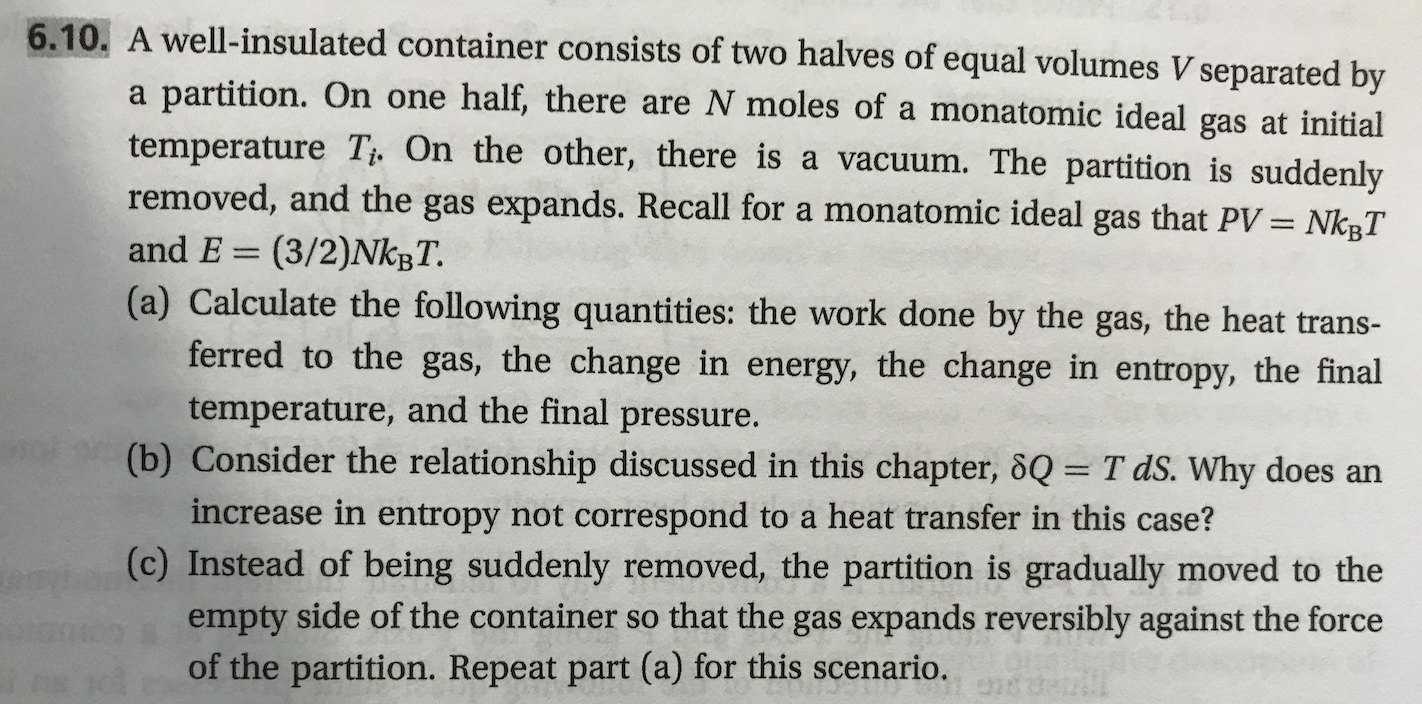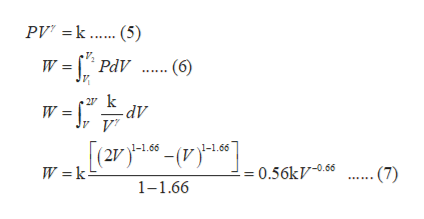# 6.10. A well-insulated container consists of two halves of equal volumes V separated bypartition. On one half, there are N moles of a monatomic ideal gas at initialtemperature Ti. On the other, there is a vacuum. The partition is suddenlyremoved, and the gas expands. Recall for a monatomic ideal gas that PV = NkgTaand E(3/2)NKBT.(a) Calculate the following quantities: the work done by the gas, the heat trans-ferred to the gas, the change in energy, the change in entropy, the finaltemperature, and the final pressure.(b) Consider the relationship discussed in this chapter, 8Q= T dS. Why do es anincrease in entropy not correspond to a heat transfer in this case?(c) Instead of being suddenly removed, the partition is gradually moved to theempty side of the container so that the gas expands reversibly against the forceof the partition. Repeat part (a) for this scenario.

Question

This problem is (6.10) from a book  "Thermodynamics and Statistical Mechanics An Integrated Approach by M. Scott Shell"help_outlineImage Transcriptionclose6.10. A well-insulated container consists of two halves of equal volumes V separated by partition. On one half, there are N moles of a monatomic ideal gas at initial temperature Ti. On the other, there is a vacuum. The partition is suddenly removed, and the gas expands. Recall for a monatomic ideal gas that PV = NkgT a and E (3/2)NKBT. (a) Calculate the following quantities: the work done by the gas, the heat trans- ferred to the gas, the change in energy, the change in entropy, the final temperature, and the final pressure. (b) Consider the relationship discussed in this chapter, 8Q= T dS. Why do es an increase in entropy not correspond to a heat transfer in this case? (c) Instead of being suddenly removed, the partition is gradually moved to the empty side of the container so that the gas expands reversibly against the force of the partition. Repeat part (a) for this scenario. fullscreen
check_circle

Step 1

Part (a): Given that the container is well insulated, N moles of a monoatomic ideal gas expands irreversibly from initial volume V1 = V to final volume V2 = 2V at an initial temperature Ti. Let initial pressure of the system be P1 and final pressure be P2.

As the given system is thermally well insulated, there will be no exchange of heat between system and surrounding. Also, no work is done on the surrounding as gas is freely expanding against vacuum. Now, according to first law of thermodynamics expressed by equation (1), since work done and heat exchange is zero, as a result change in internal energy ΔE is also zero.

For an ideal gas, change in internal energy is the function of temperature only. Therefore, the temperature of the system remains constant, making the process isothermal.

For an isothermal process involving ideal gas Boyle’s law is applicable which is represented in equation (2). Since, volume is doubled therefore, the pressure will be halved according to equation (2).

Step 2

It is known that the entropy is a state function, meaning, it depends on final and initial state of the system, rather than the path followed by it. Let us assume a reversible path that brings about the given change in volume. Entropy of the surrounding will be zero, as there is no interaction of system with the surrounding. Entropy of the system for the defined path is then given by equation (3).

Part (b): The relation for entropy represented in equation (4) is applicable for reversible processes only. Here, the free expansion of an ideal gas is an irreversible process. Therefore, entropy change do not correspond to the heat transfer in this case.

Step 3

Part (c): As the gas is expanding reversibly now in a well-insulated container, proceed by considering the process to be adiabatic. An adiabatic process follows equation (5) where k is constant....help_outlineImage TranscriptionclosePV k 5) W PdV .6) 2 k -dV V 1-1.66 1-1.66 W k - 0.56kV-0.66 (7) 1-1.66 fullscreen

### Want to see the full answer?

See Solution

#### Want to see this answer and more?

Solutions are written by subject experts who are available 24/7. Questions are typically answered within 1 hour.*

See Solution
*Response times may vary by subject and question.
Tagged in

### Chemical Engineering